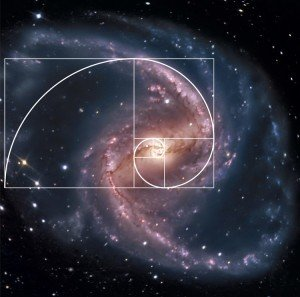# The Mathematics of the Golden RatioThe golden ratio, which is also known as the golden section, the golden mean, or the golden proportion, is a term that refers to divine proportionment, which the Greeks referred to as phi. It represents a certain ratio of elements, and is all about “relationship.”

A marvelous example of the golden ratio is found in the nautilus shell of our logo. The spirals and proportions of a nautilus present the perfect golden ratio. Its specific properties are represented in what is called a golden rectangle. (See the drawing below.)

In a golden rectangle, the ratio of the width and the length are equal to phi. (To see how this works, there is a manipulative here to play with.) If a square is created within the rectangle, the part that is cut off forms another rectangle. With the first rectangle, create a spiral from the square by drawing a 90-decree curve from sides “a” and “b” of the square, and continue doing this for the other squares, and you have the golden ratio spiral. (See photo below.)

By dividing the rectangle into smaller and smaller sections, each one approximates the golden ratio, and as you continually create successive squares and spirals out of the first rectangle, the equation of the sides approximates more closely 1.618033, which is phi or the golden ratio.If you enjoy math and want a simple explanation of the golden ratio, read here. 🙂